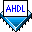## For Generate Statement

 See AlsoThe For Generate Statement lists a series of behavioral statements to be repeated.

The following example shows an iterative For Generate Statement:

```CONSTANT NUM_OF_ADDERS = 8;

SUBDESIGN 4gentst
(
)

VARIABLE

BEGIN
carry_out = cin;
FOR i IN 1 TO NUM_OF_ADDERS GENERATE
c[i] = a[i] \$ b[i] \$ carry_out[i];		% Full Adder %
carry_out[i+1] = a[i] & b[i] # carry_out[i] & (a[i] \$ b[i]);
END GENERATE;
END;```

The For Generate Statement has the following characteristics:

• The keywords `FOR` and `GENERATE` enclose the following items:

1. A temporary variable name, which consists of a symbolic name that is used only within the context of the For Generate Statement, that is, the variable ceases to exist after the Compiler processes the statement. In the example shown above, the variable is `i`. This variable name cannot be a constant, parameter, or node name that is used elsewhere in the project.

2. The word `IN`, which is followed by a range delimited by two arithmetic expressions. The arithmetic expressions are separated by the `TO` keyword. In the example shown above, the arithmetic expressions are `1` and `NUM_OF_ADDERS`. The range endpoints can consist of expressions containing only constants and parameters; variables are not required.

• The `GENERATE` keyword is followed by one or more logic statements, each of which ends with a semicolon (`;`).

• The keywords `END GENERATE` and a semicolon (`;`) end the For Generate Statement.